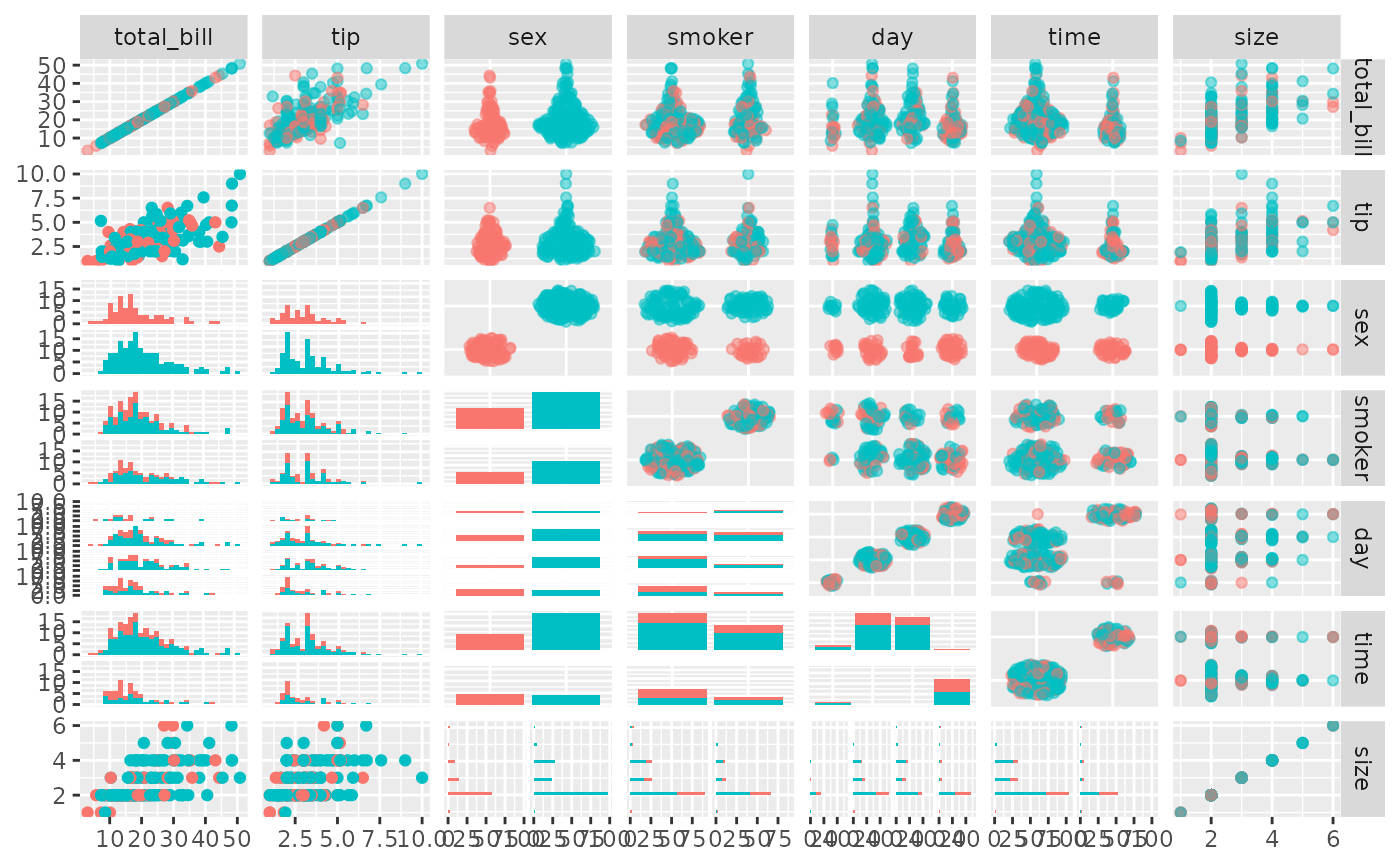Make scatterplots compatible with both continuous and categorical variables using geom_autopoint from package ggforce.

ggally_autopoint(data, mapping, ...)

ggally_autopointDiag(data, mapping, ...)

## Arguments

data data set using aesthetics being used other arguments passed to geom_autopoint(...)

## Author

Joseph Larmarange

## Examples

# Small function to display plots only if it's interactive
p_ <- GGally::print_if_interactive

data(tips, package = "reshape")
p_(ggally_autopoint(tips, mapping = aes(x = tip, y = total_bill)))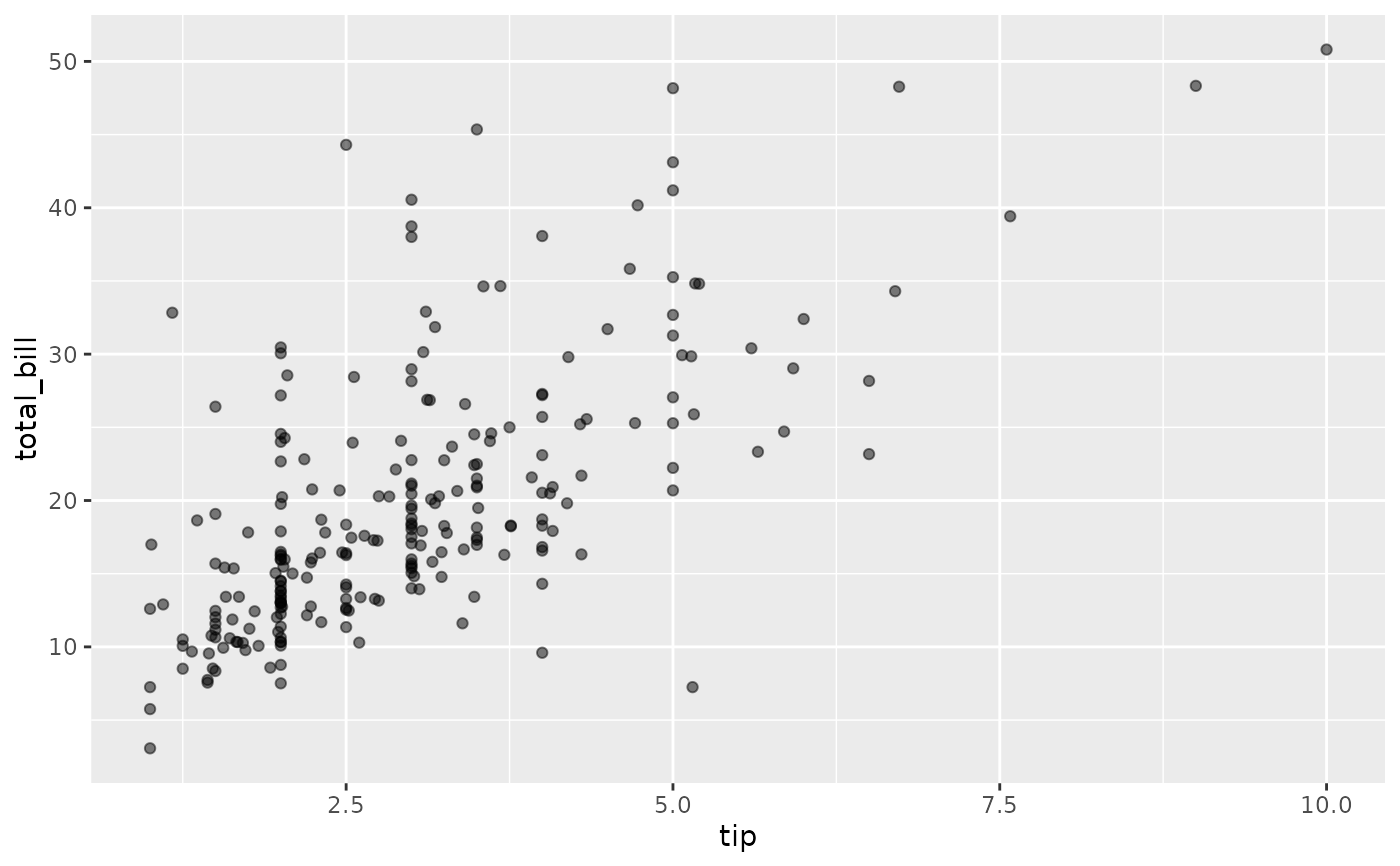p_(ggally_autopoint(tips, mapping = aes(x = tip, y = sex)))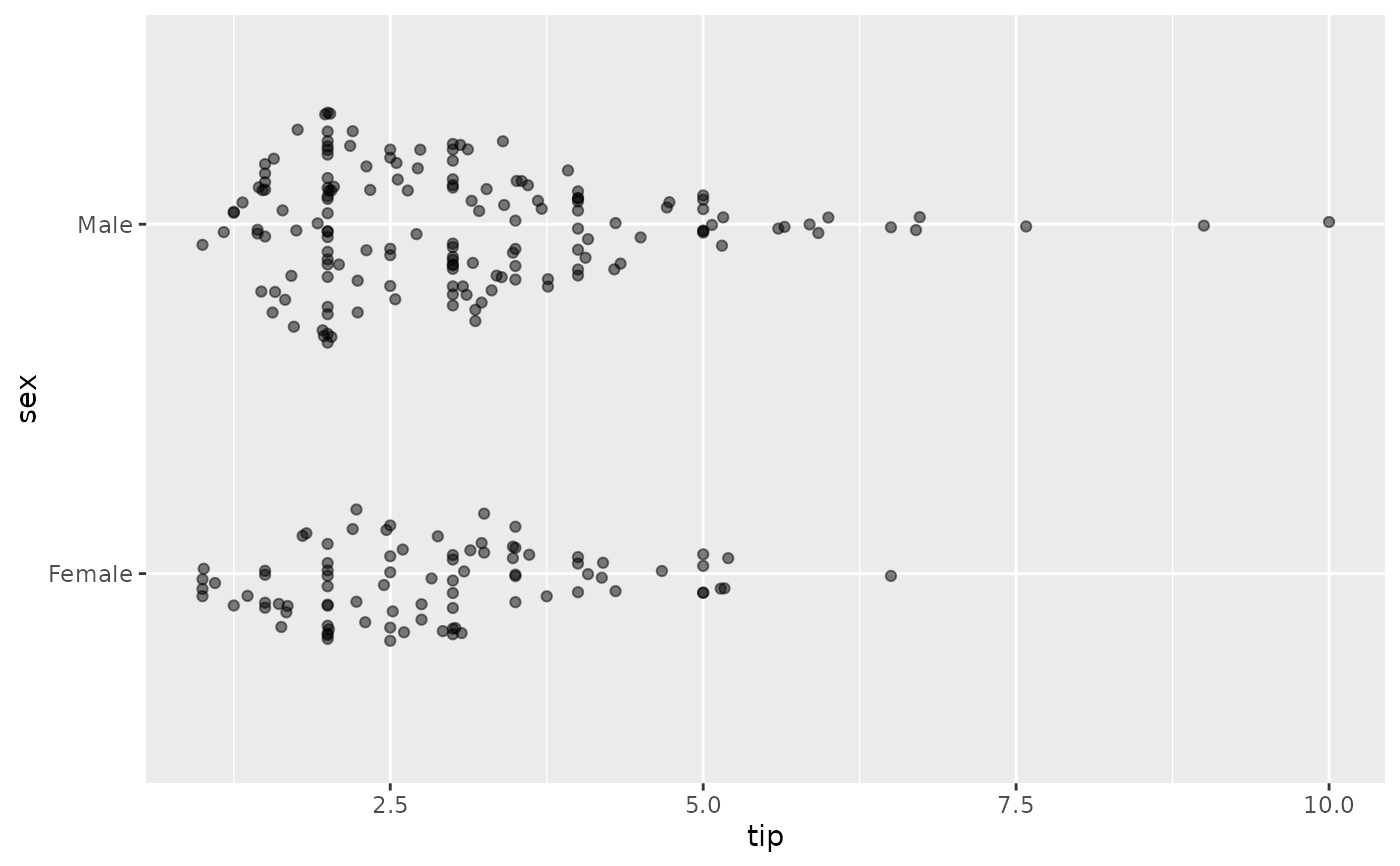p_(ggally_autopoint(tips, mapping = aes(x = smoker, y = sex)))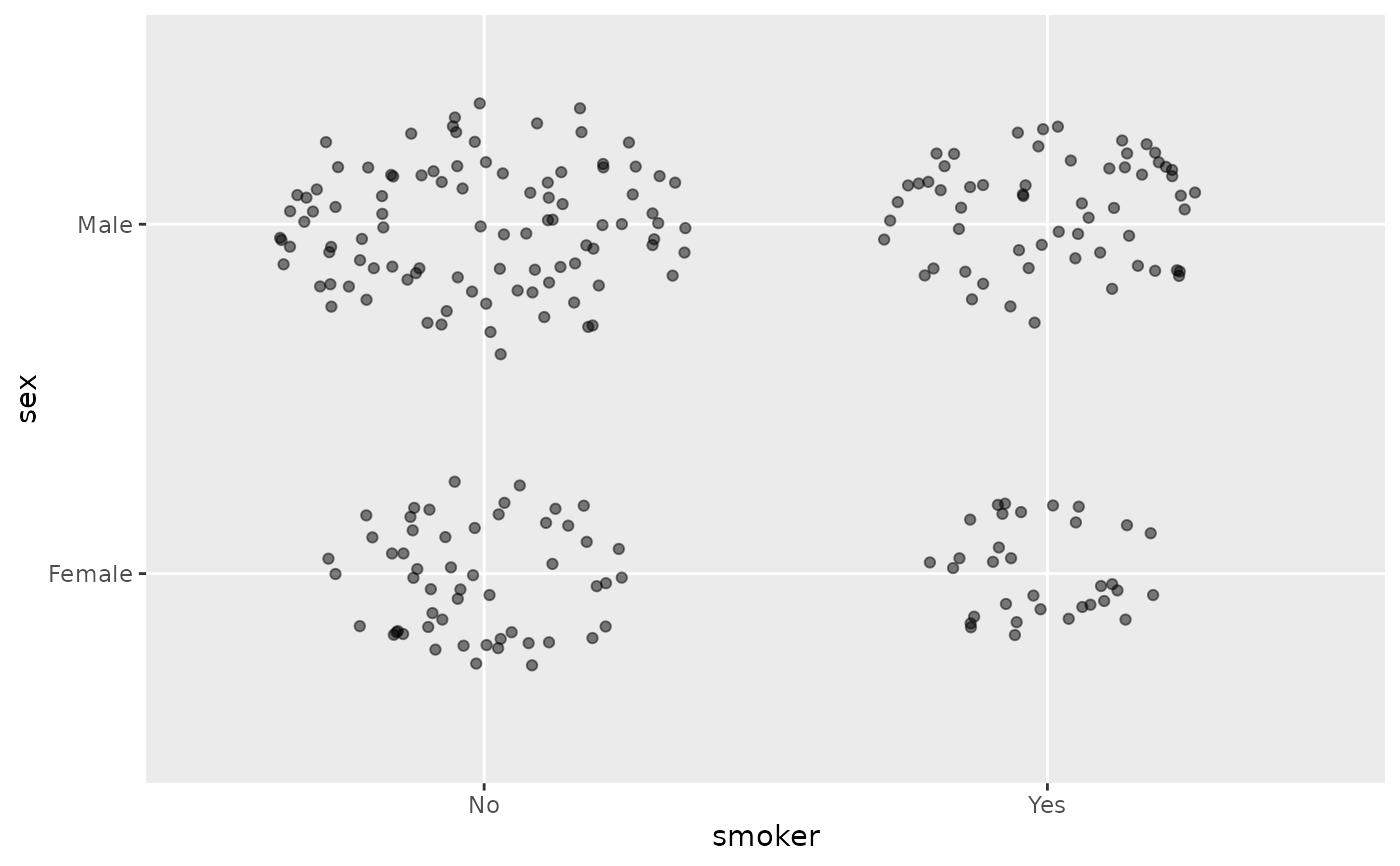p_(ggally_autopoint(tips, mapping = aes(x = smoker, y = sex, color = day)))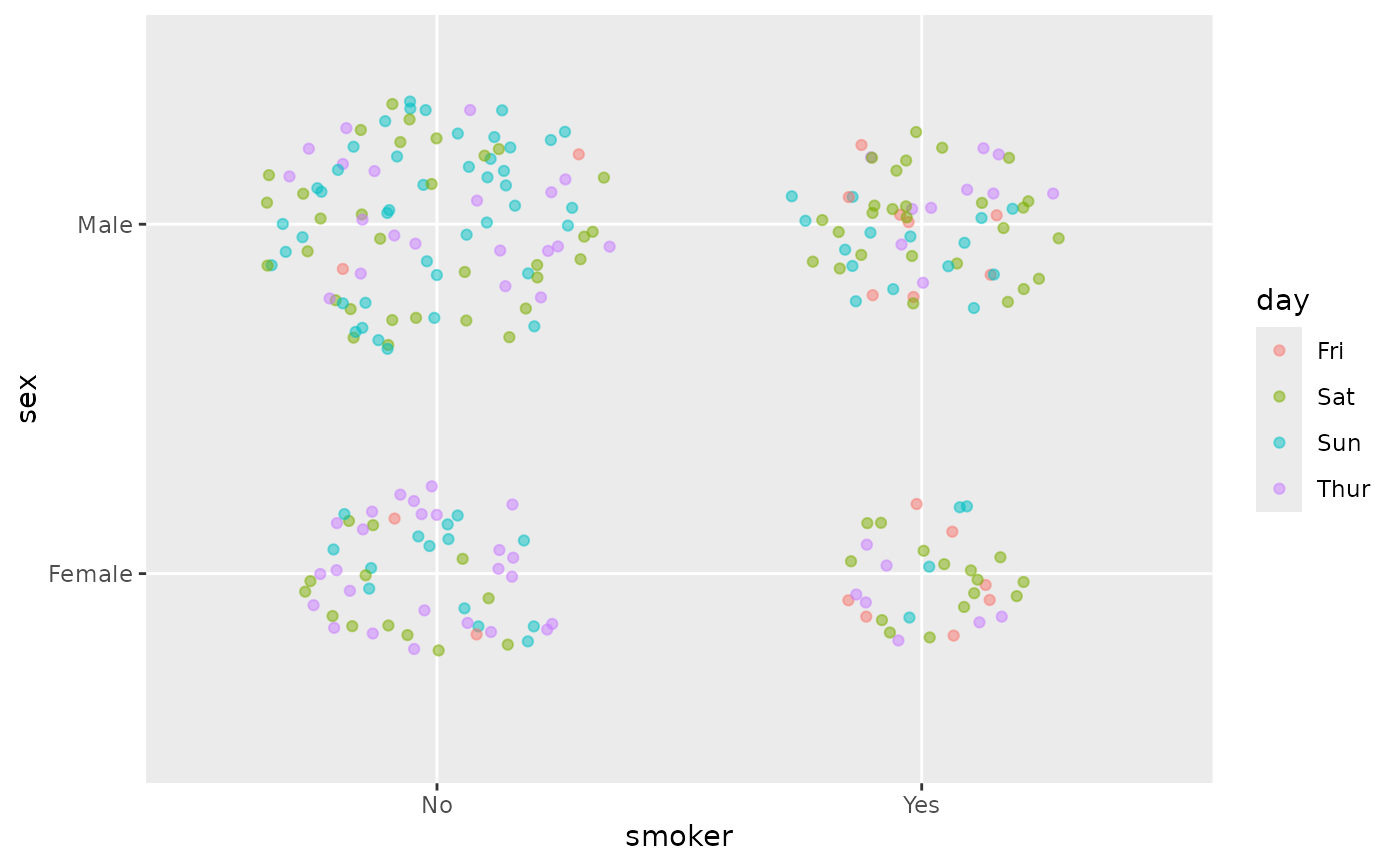p_(ggally_autopoint(tips, mapping = aes(x = smoker, y = sex), size = 8))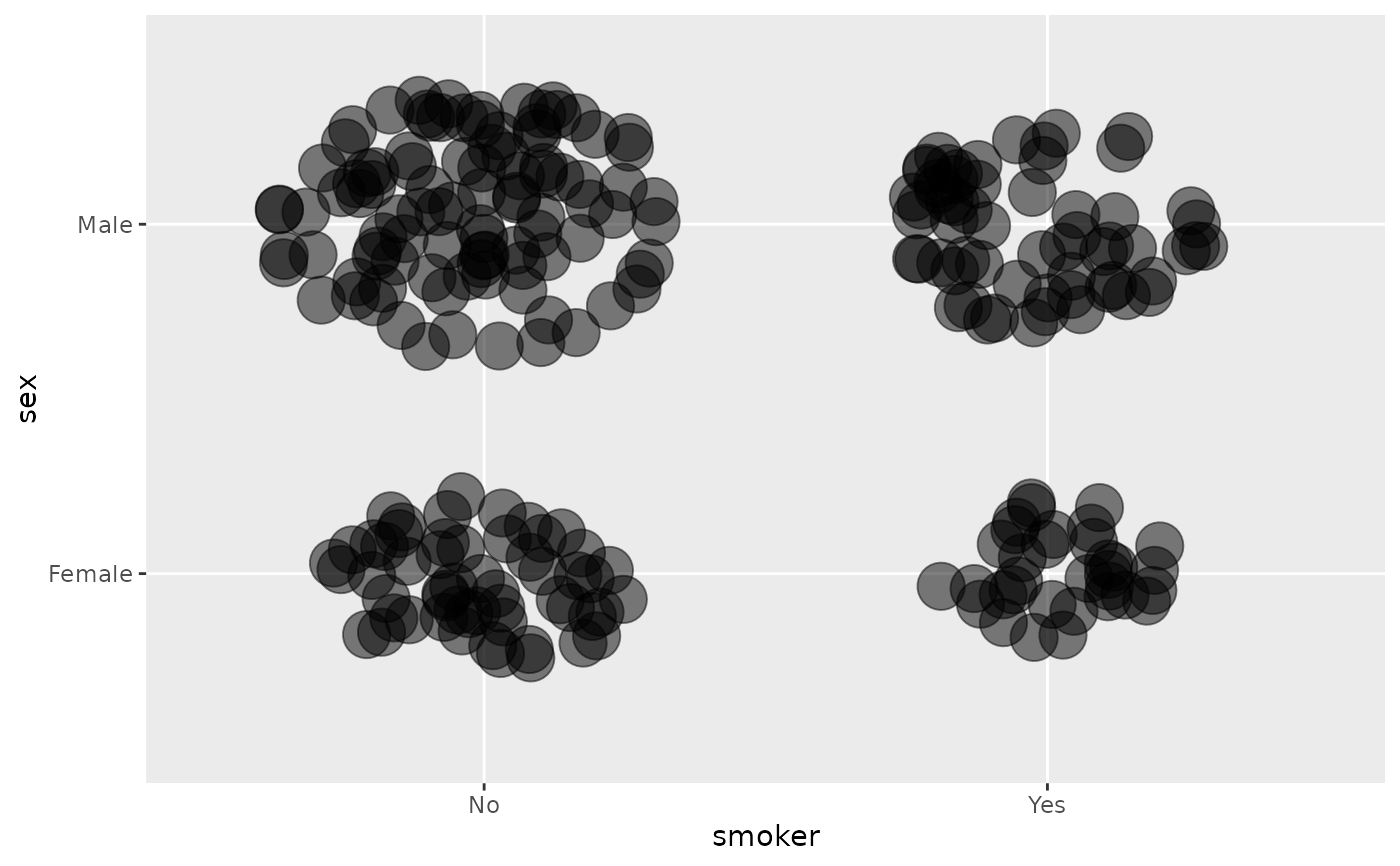p_(ggally_autopoint(tips, mapping = aes(x = smoker, y = sex), alpha = .9))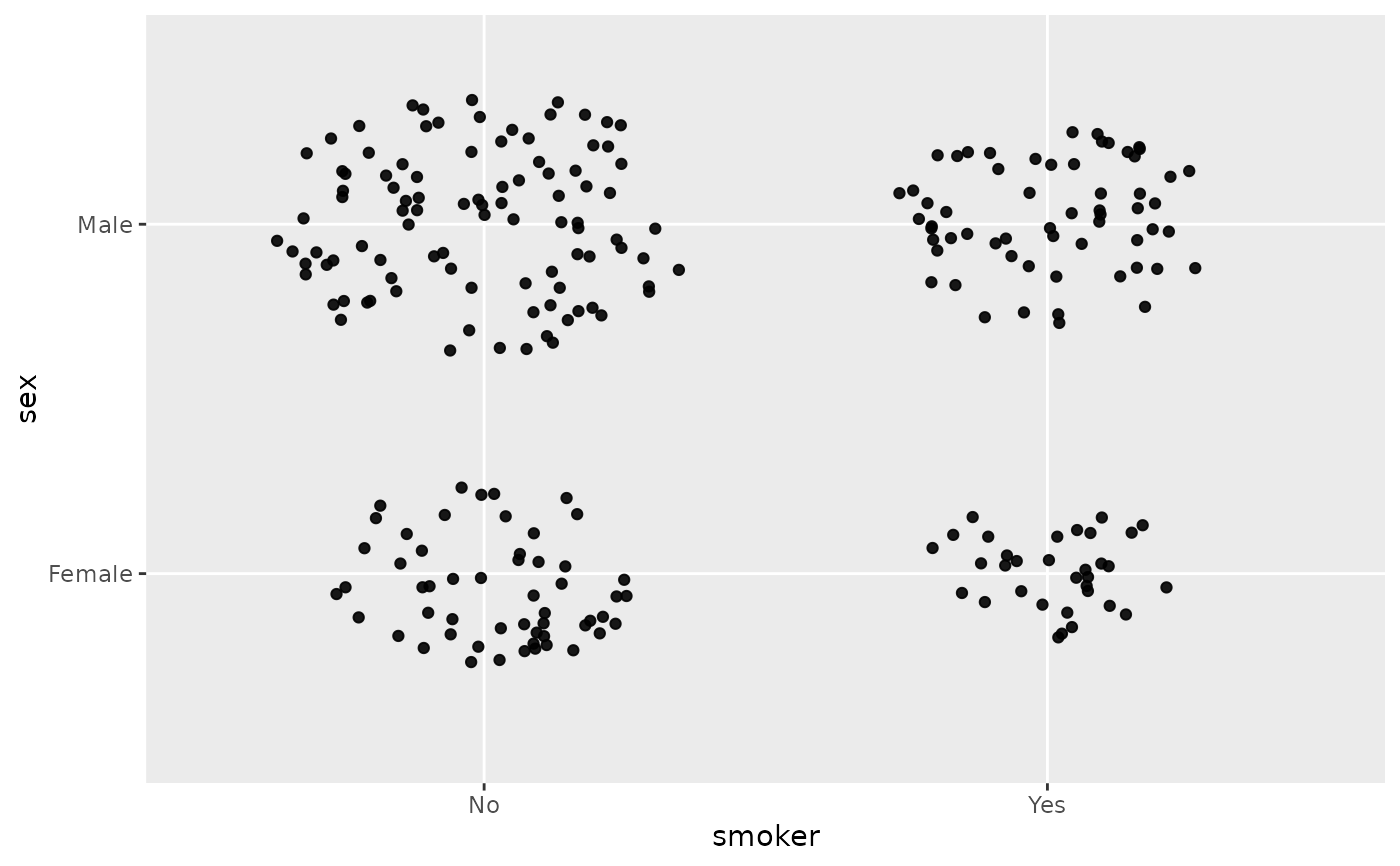p_(ggpairs(
tips,
mapping = aes(colour = sex),
upper = list(discrete = "autopoint", combo = "autopoint", continuous = "autopoint"),
diag = list(discrete = "autopointDiag", continuous = "autopointDiag")
))
#> stat_bin() using bins = 30. Pick better value with binwidth.#> stat_bin() using bins = 30. Pick better value with binwidth.#> stat_bin() using bins = 30. Pick better value with binwidth.#> stat_bin() using bins = 30. Pick better value with binwidth.#> stat_bin() using bins = 30. Pick better value with binwidth.#> stat_bin() using bins = 30. Pick better value with binwidth.#> stat_bin() using bins = 30. Pick better value with binwidth.#> stat_bin() using bins = 30. Pick better value with binwidth.#> stat_bin() using bins = 30. Pick better value with binwidth.#> stat_bin() using bins = 30. Pick better value with binwidth.#> stat_bin() using bins = 30. Pick better value with binwidth.#> stat_bin() using bins = 30. Pick better value with binwidth.